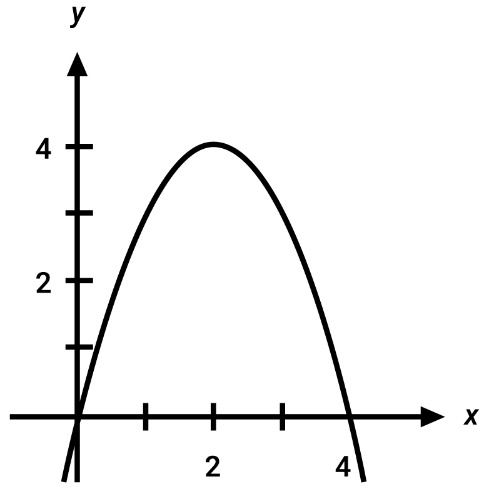Chapter 1, Problem 21REMathematical Applications for the ...

12th Edition
Ronald J. Harshbarger + 1 other
ISBN: 9781337625340

Solutions

Chapter
SectionMathematical Applications for the ...

12th Edition
Ronald J. Harshbarger + 1 other
ISBN: 9781337625340
Textbook Problem

For the function f graphedin Exercise 18, for what values of x does f ( x ) = 0 ?

To determine

The value of x such that f(x)=0 from the graph given below.Explanation

Given Information:

The graph is:

Explanation:

From the graph, the function becomes 0 at places where it crosses the x axis.

The given graph cuts the x axis at two places.

Still sussing out bartleby?

Check out a sample textbook solution.

See a sample solution

The Solution to Your Study Problems

Bartleby provides explanations to thousands of textbook problems written by our experts, many with advanced degrees!

Get Started

Find more solutions based on key concepts